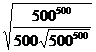Mr. Hansen’s Scavenger Hunt Questions
March 13, 2000
(abbreviations may be viewed at www.modd.net/abbrevs.htm)

#### Form III (choose any 2):

1. What is the formula for the surface area of a sphere?

2. What is Hero’s Formula for the area of a triangle in terms of the lengths of the three sides?

3. What does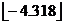equal? (Simplify your answer.)

Form IV (choose any 2):

4. The following is an erroneous proof thatCircle the step in which the error first appears, and write a concise explanation of why that step does not follow from the one before it.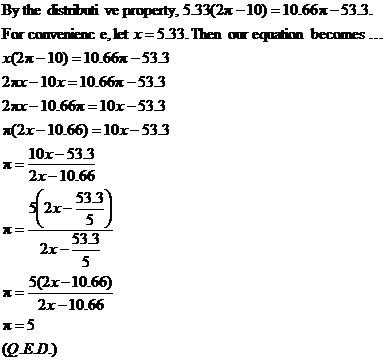5. The following is an erroneous proof that –1 = 1. Circle the step in which the error first appears, and write a concise explanation of why that step does not follow from the one before it.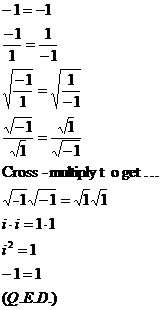6. If x = –4.656, simplify the expression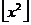.

Form V (do both, or substitute one of the Form IV problems for one of these):

7. What is the largest positive integer n for which there exists a general formula for the roots of the polynomial equation P(x) = 0, where deg(P) = n?

8. In what year was the formula described in #7 first published?

#### Form VI (do both, or substitute one of the Form IV or Form V problems for one of these):

9. For what values of x, if any, is the cube root of x3 equal to the square root of x2? State your answer as a solution set.

10. Find the value of the following expression to the nearest power of ten (show your work):